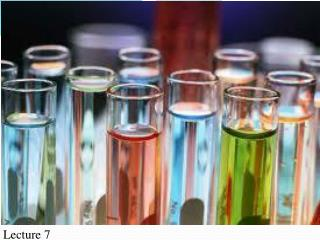Download PresentationIntroduction to Analytical Chemistry

Introduction to Analytical Chemistry - PowerPoint PPT Presentation

Introduction to Analytical Chemistry. Dr M. Abd-Elhakeem . Faculty of Biotechnology General Chemistry Lecture 7. What is Analytical Chemistry?.I am the owner, or an agent authorized to act on behalf of the owner, of the copyrighted work described.
Download PresentationIntroduction to Analytical Chemistry

Download Policy: Content on the Website is provided to you AS IS for your information and personal use and may not be sold / licensed / shared on other websites without getting consent from its author.While downloading, if for some reason you are not able to download a presentation, the publisher may have deleted the file from their server.

- - - - - - - - - - - - - - - - - - - - - - - - - - E N D - - - - - - - - - - - - - - - - - - - - - - - - - -
Presentation Transcript
1. Introduction to Analytical Chemistry Dr M. Abd-Elhakeem Faculty of Biotechnology General Chemistry Lecture 7

2. What is Analytical Chemistry? • Analytical chemistry is the study of the separation, identification, and quantification of the chemical components of natural and artificial materials

3. What is Analytical Chemistry? Separation ------------ Analyses

4. Separation : convert a mixture of substances into two or more distinct product. Separations are carried out based on differences in physical or chemical properties such as size, shape, mass, or chemical affinity between the constituents of a mixture.

5. 2- Analysis • Qualitative Analysis • Quantitative Analysis

6. Qualitative analysis • The determination of the components of an unknown sample.

7. Quantitative analysis • The determination of the quantity of the components in a sample.

8. Forensic Science Medicine Analytical Science Biochemistry Materials Science Organic Chemistry Analytical Chemistry is a central science

9. Vocabulary • Accuracy • Precision • Analyte • Blank • Concentration • Calibration • Standard Addition • Standardisation • Detection Limit • Interference • Internal Standard • Masking • Matrix

10. Standard • Sample • Primary Standard • Reagent • Sensitivity • Method • Technique • Validation

11. Method: is the technique that used to extract, determine the type and the concentration of the analyte.

12. Accuracy: • The accuracy of an analytical measurement is how close a result comes to the true value. Determining the accuracy of a measurement usually requires calibration of the analytical method with a known standard. Precision • Precision is the reproducibility of multiple measurements and is usually described by the standard deviation, standard error.

13. Identify 1- Analyte 2- Sample matrix 3- Standard 4- Interference 5- Blank 6- Masking agent Sample for chemical analysis of

14. Analyte: is a substance or chemical constituent that is of interest in an analytical procedure Standard Standards are materials containing a known concentration of an analyte. They provide a reference to determine unknown concentrations or to calibrate analytical instruments.

15. Primary standard: • A primary standard is a reagent that characterize by • High purity • Stability (low reactivity) • Low hygroscopic (hydrophobic) • High solubility (if used in titration)

16. Calibration • Making measurements with any analytical method or instrument requires calibration to ensure the accuracy of the measurement. There are two common calibration procedures: using a standard curve, and the standard-addition method.

17. Standard curve

18. Concentration: is the amount of a constituent in a known amount of mixture matrix.

19. masking agent: is a reagent used in chemical analysis which reacts with chemical species that may interfere in the analysis. Sensitivity: of a chemical analysis method is the ability to detect the minor amount of the analyte without interference.

20. Sample matrix: refers to the components of a sample other than the analyte. The matrix can have a considerable effect on the way the analysis is conducted and the quality of the results obtained. Detection limit: It is the lowest quantity of a substance that can be distinguished from the absence of that substance.

21. Blank: A blank solution is a solution containing no analyte of interest, usually used to calibrate instruments • Interference: An interference is a substance, other than the assayed material, that can be measured by the chosen analytical method or that can prevent the assayed material from being measured.

22. Validation:Method validation is the process used to confirm that the analytical procedure employed for a specific test is suitable for its intended use. It include Determination of Detection limit Accuracy Precision Linearity Sensitivity specificity

23. ECRIQ • Identify the problem: translate general questions into specific questions amenable to being answered through chemical measurements. • Selecting procedures Sampling • Sample preparation • Obtain a representative bulk sample from the lot. • a- Extract from the bulk sample a homogeneous laboratory sample.

24. b- Convert the laboratory sample into a form suitable for analysis, which usually means dissolving the sample. Samples with a low concentration of analyte may need to be concentrated prior to analysis. • c- Remove or mask species that interfere with the chemical analysis. • d- Identify and Quantify the analyte in several aliquots and also characterize the structure of the unknown compounds • Reporting and interpretation